## Mathematics Paper 2 Questions and Answers - Bunamfan Cluster Pre Mock Exam 2022

INSTRUCTIONS TO CANDIDATES

• The paper contains two sections: Section I and II
• Answer ALL questions in section I and ANY FIVE questions from section II.
• All working and answers must be written on the question paper in the spaces provided below each question.
• Marks may be awarded for correct working even if the answer is wrong.
• Negligent and slovenly work will be penalized.
• Non-programmable silent electronic calculators and mathematical tables are allowed for use.## Questions

SECTION 1 (50marks)
Answer all questions in this section

1. The length and width of a rectangle were measured as 12.4cm and 5.0cm respectively. Find to 4 significant figures, the percentage error in calculating the area of the rectangle (3mks)
2. The co-ordinates of a point A is (2, 8, 3) and B is (-4, -8, -5). A point P divides AB externally in the ratio 7: 3. Determine the co-ordinates of P (3mks)
3. Solve for x:
Solve for x: (log3x)2 – ½ log3 x= 3/2 (3mks)
4. A circle is tangent to the y – axis and intersects the x- axis at (2,0) and (8,0). Obtain the equation of the circle in the form x2 + y2 +ax +by +c = 0, where a, b and c are integers (4mks)
5. Solve for X in the equation:
2 Sin2x – 1 = Cos2x + Sin x, for 00 ≤ x ≤ 3600 (3mks)
6. Make P the subject of the formula (3mks)
T=³√(P²+n) + R
m2
7. The figure below represents a flower garden ABC. A tap T is to be placed inside the triangle such that it is nearer A than B and angle ATB is greater than 120°. By construction, show the region T where the tap can be positioned. (4mks)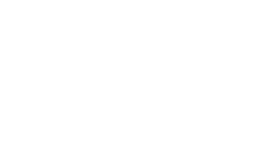8. PQRS is a rectangle whose area 170cm2. The internal rectangle measures (x+5)cm by (x-2)cm.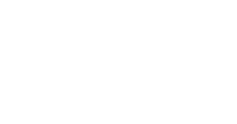Determine the area of the shaded part if the thickness of this part is 0.1x cm (4mks)
9. Find the length of an arc of a circle which subtends an angle of 0⋅8 radians at the center of the circle. The radius of the circle is 15 cm. (2mks)
10. A map has a scale of 1: 25 000. On this map a square piece of land is represented by an area of 2cm2. Calculate the actual area in hectares of the land. (2mks)
11. A dealer has two types of grades of tea, A and B. Grade A costs shs.140 per kg while grade B costs shs.160 per kg. Determine the ratio he should mix A and B so that he makes a 60% profit when he sells the mixture at shs.232 per kg. (3mks)
12. The data below shows marks scored by 8 form four students in Molo district mathematics contest44, 32, 67, 52, 28, 39, 46, 64. Calculate the mean absolute deviation of the data. (3mks)
13. A variable y varies as the square of x and inversely as the square root of z. Find the percentage change in y when x is changed in the ratio 5:4 and z reduced by 19% (3mks)
14. Simplify 2√2/1+√2√2/1-√2 = a + b√c leaving your answer in the form a + b√c, where a, b and c are rational numbers. (3mks)
15. When a man is standing at a point x, he observes that the angle of elevation of the top of a flag post is 13°, he walks 120 m towards the flag post and the angle of elevation is 30°. If the eyes of the man are 1.5m from the ground, find the height of the flag post. (4mks)
16. Find the value of x given that T is a singular matrix. (3mks)
T = x+1 2 4x 2x

SECTION II 50 marks
(Answer only five questions in this Section)

1. A tank has two water taps P and Q and another tap R. When empty the tank can be filled by tap P alone in 5 hours or by tap Q in 3 hours. When full the tank can be emptied in 8 hours by tap R.
1. The tank is initially empty. Find how long it would take to fill up the tank
1. If tap R is closed and taps P and Q are opened at the same time. (2mks)
2. If all the three taps are opened at the same time.Giving your answer to the nearest minute. (2mks)
2. Assume the tank initially empty and the three taps are opened as follows;
P at 8:00 am, Q at 9:00 am and R at 9:00 am
1. Find the fraction of the tank that would be filled by 10:00 am. (3mks)
2. Find the time the tank would be fully filled up. Give your answer to the nearest minute. (3mks)
2. In the figure below, G is the center of the circle. <DCB = 50˚, <AGB = 63˚ and <FEC = 111˚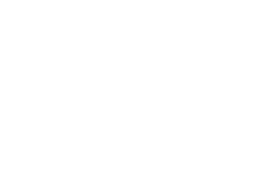1. Determine the size of the following angles
1. FAC (1mk)
2. BAC (1mk)
3. ACB (1mk)
4. ACF (1mk)
5. AFC (1mk)
2. DC is a tangent to the circle at C and its length is 6cm. AD is a straight line and chord AB is 3.1cm. Determine
1. The length of BD (2mks)
2. Area of triangle ABC (3mks)
3. The table below shows the income tax rates in a certain year.
 Annual taxable income in Kenya shillings Tax rate in % 0 - 144000 0% 144 001 - 300 000 10 300 001 - 468 000 15 468 001 - 648 000 20 648 001 - 840 000 25 Above 840 000 30
During that year Kurenta’s annual gross tax in sixth band was Shs 108 000.
1. Determine Kurenta’s annual gross tax. (3mks)
2. If he enjoyed annual relief of Shs. 21 000, determine his monthly net tax (P.A.Y.E)(2mks)
3. Kurenta had a basic salary of Shs X Shs p.a. and enjoyed non-taxable allowances that is equivalent to 45% of basic salary. Determine Kurenta’s gross salary p.m. (3mks)
4. The following deductions were also made from Kurenta’s salary every month: Co-operative shares 8 000, Co-operative loans 12 000, Pension scheme 4 000, Union dues 2000. (2mks)
Determine Kurenta’s monthly net salary during that year.
4.
1. Complete the table below for the functions y=-52+92cos2x and y=3sin7x3to 1 d.p.for O0≤ x ≥ 1650 (2mks)
 x 0 15 30 45 60 75 90 105 120 135 150 165 y = -⁵/₂ + ⁹/₂Cos(2x) 2 1.4 -2.5 -4.8 -6.4 -7 -6.4 -2.5 1.4 y = 3Sin(7x/3) 0 1.7 2.8 2.9 1.9 -2.7 -2.9 -2.1 1.3
2. Draw the graphs of y= -5/+ 9/2cos2x and y=3sin(7x/3) on the same grid.
(use a scale of 1cm to rep 100on the x-axis and 1cm to rep 1unit on the y-axis.) (5mks)
3. Use your graph to solve 9cos2x - 6sin(7x/3)=5 (3mks)
5. The figure below shows a cuboid ABCDEFGH with AB = 8cm, BC = 6cm and CH = 5cm.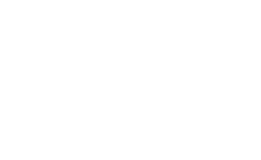Calculate to 1 d.p
1. The length BE (2mks)
2. The angle between BE and plane ABCD (1mk)
3. The angle between lines FH and BC. (2mks)
4. The acute angle between lines FH and BD (1mks)
5. The angle between place AGHD and planes ABCD (2mks)
6. Point K and L divides EH and FG respectively in the ratio 1: 3. Determine the angle between planes ADKL and BCKL (2mks)
6. Juice type Q is 60% tangerine and the rest is water. Juice type P is 30% water and the rest is pineapple.
1. Jane mixes 8 litres of Q with 12 litres of P to produce a blend juice B. Find the percentage of water in blend B (2mks)
2. Janet makes 8L of a blend juice R that is 36% water by mixing juice Q and P. Determine the amount of juice Q and P that she uses. (4mks)
3. 5L of B is now mixed with 3L of Q to produce blend W. Find the concentration of tangerine in blend W. (4mks)
7. In triangle OAB below OA = a, OB = b point M lies on ON such that OM: MA= 2:3 and point N lies on OB such that ON = 5/6OB line AN intersect line MB at X.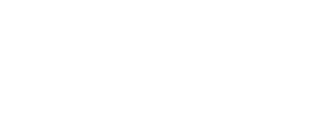1. Express in terms of a and b
1. AN (1mk)
2. BM (1mk)
2. Given that AX=kAN and BX=rBM where k and r are scalars.
1. write down two different expression for OX in terms of a,b,k and r. (2mks)
2. Find the value of k and r. (4mks)
3. Determine the ratio in which x divides line MB. (2mks)
8. The probability that it rains on a certain day is 0.8. If it rains the probability that Onguti comes to school late is 0.7 but otherwise it is 0.4. If he comes to school late, the probability that he fails an exercise is 0.8 but if he comes early, the probability of failing an exercise is 0.3
1. Draw a tree diagram to represent this information (2mks)
2. Determine
1. The probability that it rains, he comes to schools early and he fails the exercise (2mks)
2. The probability that he passes his exercise (2mks)
3. The probability that he comes to school late (2mks)
4. The probability that he comes to schools late and he passes his exercise (2mks)## Marking Scheme

1. W.A = 12.4 x 5.0 = 62
Max A = 12.45 x 5.05 = 62.8725
Min A = 12.35 x 4.95 = 61.1325
A.E = 62.8725 - 61.1325 = 0.87
2
0.87   x 100
62
= 1.403%
2.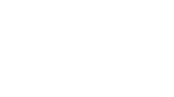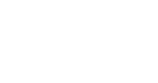3. Let Log3x be a
a2 - 1/2a = 3/2
2a2 - a - 3 =0
(a+1)(2a-3)= 0
a=-1 , 1=3/2
Log3x =-1
x = 3-1= 1/3
Log3x = 1.5
x = 31.5 = 5.196
x=1/3 & 5.196
4.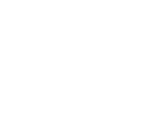center (5,4) r =5
(x-5)2 + (y-4)2 = 52
x2 - 10x + 25 + y - 8x = 16 = 25
x2 + y2 - 10x - 8x + 16 = 0
5. Cos2x = 1 - Sin2x
2Sin2x - 1= 1 - Sin2x + Sinx
3Sin2x - Sinx - 2=0
3a2 - a -2 = 0
(3a+2)(a-1)=0
a= -2/3 and a=1
Sin x = -2/3      Sin x =1
Sin-1 2/3 = 41.81°    x =90°
x= 221.81 , 318.19
x=90°, 221.81°, 318.19°
6. [] = (³√(P² + n))3
m²
P² + n
m²
P² + n = m² [T-R]3
P² = m² [T-R]3 -n
P = √(m² [T-R]3 -n)
7.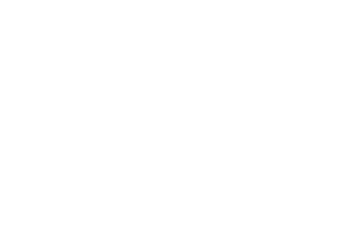8. 1(1.2x + 5)(1.2x -2)= 170
1.44x² + 3.6x - 180 = 0
2x² + 5x - 250 =0
x = -5 ± √(25 + 2000)
4
x = -12.5 , x= 10
(10+5)(10-2)
= 170 - 120= 50cm2
9. L/15= 0.8
L= 12cm
10. A.S.F = 1:25 000
Actual area = 25000² x 2
10 000
125000m2
125000
10000
12.5Ha
11. Cost = 232/160 x 100 = 145
140A + 160B = 145
A +B
140A + 160B = 145A + 145B
15B = 5A
A/B = 3/1
A:B
3:1
A:B
140:160
145 → 15:5
12. mean = 44 + 32 + 67 + 52 + 28 + 39 + 46 + 64 = 46.5
8
d = -2.5, -14.5 , 20.5 , 5.5, -18.5 , -7.5, -0.5, 17.5
Σ|d| = 2.5 + 14.5 + .....+ 17.5
N                   8
= 10.875
13. y α x²/√Z
y = Kx²√Z
x1 = 5/4x = 1.25x
Z1 =0.81Z
y =    K(1.25x)²
√0.81Z
= 1.5625     kx²
0.9        √Z
y1 = 1.736111
% = [1.736111 - 1] x 100 = 73.61%
Z increases by 73.61%
14.       2√2        √2        =    2√2[1- √2]- √2[1+√2]
1+√2              1-√2                   1²-[√2]²
2√2 - 4 - √2 - 2
1 - 2
15.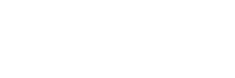Tan 13 = h/x
Tan 30 = h/x-120
xtan13 = tan 30(x -120)
x =         120 tan30
tan 30 - Tan 13
= 199.96m
h = 199.96 tan 13
h = 46.16
height = 46.16 + 1.5
47.66m
16. 2x[x+1] - 8x = 0
2x² + 2x - 8x=0
2x² - 6x = 0
x² - 3x = 0
x[x-3]=0
x=0 or x=3
17.
1.
1. 1/5 + 1/3 = 8/15
Time = 15/8 = 17/8 hrs
Accept 1.875
2. 8/15 - 1/8 = 49/120
222/49 hrs
2hrs 27mins or 147 mins
2.
1. [1/5 x 2]+ [1/3 x 1]+ [1/8 x 1]
2/5 + 1/3 + 1/8
= 73/120
2. Remaining = 1 - 73/120 = 47/120
1hour = 49/120
x = 47/120
x = 47/120 x 120/49
57.55mins ≈ 58
10:58am
18.
1.
1. 180 - 111 = 690
2. <DCB = <BAC = 50°
3. <ACB = 1/2 <AGB
1/2 x 63 = 31.5
4. ACB = 180 - (69 + 50) =  61
ACF = 61 - 31.5 = 29.5
5. 180 - (69 + 29.5) = 81.5
2.
1. x(3.1 + x) = 6.12
x2 + 3.1x = 37.21
x2 + 3.1x - 37.21=0
-3.1 ± √9.61 + 148.84
2
-3.1 ± 12.59
2
x = -7.845 & x = 4.745
BD= 4.745
2.    3.1     =      BC
Sin31.5          Sin 50
BC = 3.1Sin50  = 4.545
Sin 31.5
1/2 x 4.545 x 3.1 Sin 98.5 = 6.967
19.
1. 1st band = 0
2nd 10/100 x 156, 000= 15, 000
3rd 15/100 x 168,000 = 25, 200
4th 20/100 x 180,000 = 36, 000
5th 25/100 x 192, 000 = 48, 000
6th = 108, 000
15600 + 25200 + 36000 + 48000 + 108000 = 232800
2. 232800 - 21000
12
= 17650
3. 6th band = 30/100 x X = 108,000
x = 360,000
Taxable income = 360, 000 + 840,000 = 1, 200, 000
145/100 x 1,200,000
1,740,000
12
= 145,000
1. Sal 145000 - [17650 + 8000 + 12000 + 4000 + 2000]
= 101, 350
20.
1.
 x 0 15 30 45 60 75 90 105 120 135 150 165 y = -⁵/₂ + ⁹/₂Cos(2x) 2 1.4 -0.3 -2.5 -4.8 -6.4 -7 -6.4 -4.8 -2.5 -0.3 1.4 y = 3Sin(7x/3) 0 1.7 2.8 2.9 1.9 0.3 -1.5 -2.7 -2.9 -2.1 -0.5 1.3

2.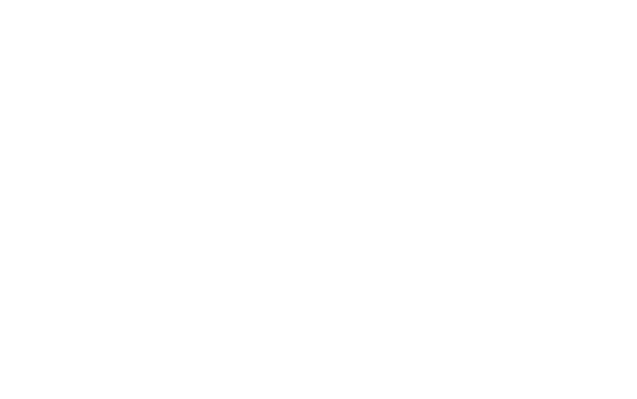3.  9Cos 2x - 6Sin 7x/3 = 5
-5 + 9Cos2x = 6 Sin 7x/3
-5/2 + 9/2 Cos2x = 6/2 Sin 7x/3
-5/2 + 9/2(0)2x = 3Sin7x/3
x=13° , x = 142°
21.
1. BD = √82 + 62 = 10cm
BE = √102 + 52 = 11.18, 11.2
2. TanQ = 5/10
Q= 26.57°
Q= 26.6°
3. Translate BC to GH
Tan Q = 8/6 = 4/3
Q = 53.13
Q = 53.1°
4. Sin 1/2 Q = 3/5
1/2 Q = Sin-1 3/5 = 36.87
Q = 36.87 x 2 = 73.74°
Q = 73.7°
5. Tan Q = 5/8
Q = Tan-1 5/8
Q = 32.01°
Q= 32.0°
6. Tan Q1 = 2/5
7. Tan Q2 = 6/5
21.80 + 50.19
71.99°
72.0°
22.
1. Tital mix = 12 + 8 = 20
water = [40/100 x 8 + 30/100 x 12] = 6.8
6.8 x 100  = 34%
20
2. 40%Q + 30%P = 36%
Q + P
40Q + 30P = 36Q + 36P
4Q = 6P
Q/P = 3/2
Q:P = 3:2
Q= 3/5 x 8 = 4.8L
P = 2/5 x 8 = 3.2L
3. Tangerine Q = 60/100 x 3 = 1.8L
% of Tangerine in B = 0.6 x 8 x 100 = 24%
20
Tangerine in 5L of B = 24/100 x 5 = 1.2L
1.8 + 1.2 x 100
8
37.5%
23.
1.
1. AO + ON
= -a + 5/6b
2. BO + OM
-b + 2/5a
2.
1. OX = OA  + AX
= a + k (-a + 5/6b)
=(1-k)a + 5/6kb
OX = OB + BX
= b + r(-b + 2/5a)
=(1-r)b + 2/5ra
2. (1-K)a + 5/6Kb = (1-r)b + 2/5ra
1-k = 2/5r
5/6k = 1-r
k= 1-2/5r
5/6(1-2/5r)= 1-r
r= 1/4
k = 1-2/5 x 1/4
k = 9/10
r = 1/4 & k = 9/10
3. 1-r:r
3/4 : 1/4
3:1
24.
1.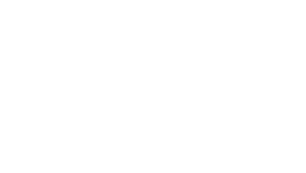2.
1. P(RL'F)
0.8 x 0.3 x 0.3 = 0.072
2. P(RLF') or P(RL'F') or P(R'LF') or P(R'L'F')
(0.8 x 0.7 x 0.2)+(0.8 x 0.3 x 0.7)+(0.2 x 0.4 x 0.2)+(0.2 x 0.6 x 0.7) = 0.38
3. P(RL) or P(R'L)
= (0.8 x 0.7) + (0.2 x 0.4)
= 0.64
4. P(ELF') or P(R'LF')
(0.8 x 0.7 x 0.2) + (0.2 x 0.4 x 0.2) = 0.128

• ✔ To read offline at any time.
• ✔ To Print at your convenience
• ✔ Share Easily with Friends / Students